The Product Rule: Solutions

1.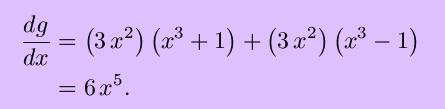If the expression for g(x) is first mulitplied out, showing that g(x) = x6-1, the same result follows immediately.

2. Let k(x)  = g(x)h(x), so that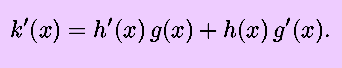Then, using the product rule again, and supressing the dependence on the variable x,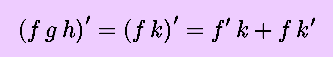and substitution for k(x) in terms of g(x) and h(x) gives the result.

Letting g(x) =  h(x) = f(x) leads to the corollary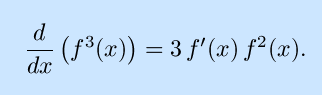3. Given:The result of the above exercise gives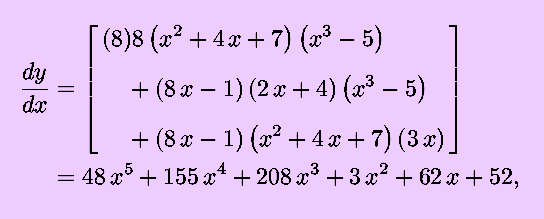and it is quite questionable whether the last expansion is worth the effort; in fact, expanding the original function and then differentiating should lead to the same result. You are encouraged to check this.

Back to the Product Rule | Back to the Calculus page | Back to the World Web Math top page

watko@mit.edu